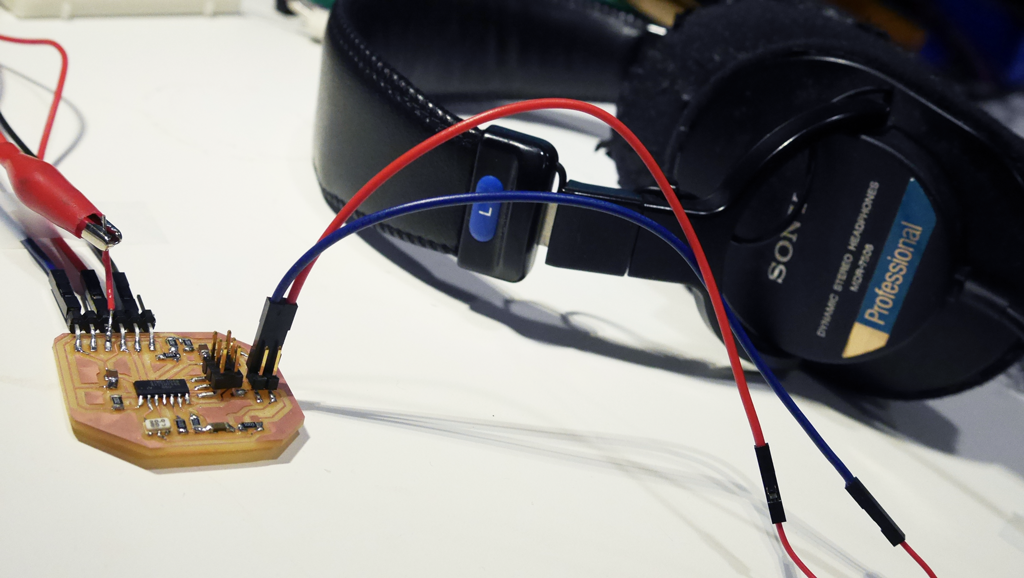How to Make (almost) Anything

12 Interface & Application Programming

I used my ATtiny44 effector circuit to program actual guitar effects this week. I fixed some problem with the previous assignment by configuring timers correctly - greatly reduces noise. Putting a capacitor at the output stage also helps achieving correct sound, as output signal is a combination of two PWM signals - needs interpolation to get the right sound.The main code:

//input
unsigned char inputLo, inputHi;
int inputRaw;
unsigned short inputRawShort;
unsigned char inputRawByte;
unsigned short output;

//effect
int fx;
int j, value50, value300, value10000, delayed;

//chorus
unsigned char buffer;
byte Progress;
boolean Direction;
byte Offset;
byte Position;
unsigned short SinceNudge;
unsigned short SinceUpdate;
unsigned short NudgeLength;

//audio processing
int iw;
byte bb;
int icnt;
int iw1;

volatile byte Timewaster;

void setup()
{
CLKPR = (1 << CLKPCE);
CLKPR = (0 << CLKPS3) | (0 << CLKPS2) | (0 << CLKPS1) | (0 << CLKPS0);

TCCR0A = ((0 << COM0A0) | (1 << COM0A1) | (0 << COM0B0) | (1 << COM0B1) | (1 << WGM01) | (1 << WGM00));
TCCR0B = (1 << CS00); // set timer 0 prescalar to 1

pinMode( 8, OUTPUT);
pinMode( 7, OUTPUT);
analogWrite( 8, 0 );
analogWrite( 7, 0 );

ADMUX = (0 << REFS1) | (0 << REFS0) // 5V ref
| (1 << MUX5) | (1 << MUX4) | (0 << MUX3) | (0 << MUX2) | (0 << MUX1) | (1 << MUX0);

ADCSRB = (1 << BIN); // bipolar mode

/* Give it four cycles to sink in. */
Timewaster++;
Timewaster--;
Timewaster++;
Timewaster--;

//init value
j = 50;
value50 = 50;
value300 = 300;
value10000 = 10000;
delayed = 0;

Progress = 0;
Direction = 0;
Offset = 0;
SinceNudge = 0;
SinceUpdate = 0;
NudgeLength = 0;
}

void loop()
{
fx = 100;

/*
HERE THE EFFECTS GO
*/

}

/*
I/O for full scale int
*/
void outputInt(int val)
{
output = val + 32768; /* Scale it up to full 16-bit. */

analogWrite( 8, output >> 8 );
analogWrite( 7, output & 255 );
}

{
/* get input */
delay(2); // Wait for Vref to settle

// rearragne bits
inputRaw = 256*inputHi + inputLo;
if (inputRaw > 511){
inputRaw -= 1024;
}
inputRaw*=64;
}

/*
I/O for unsigned short
*/
void output10(unsigned short val)
{
output = val<<6;

analogWrite( 8, output >> 8 );
analogWrite( 7, output & 255 );
}
{
/* get input */
delay(2); // Wait for Vref to settle

// rearragne bits
inputRawShort = 256*inputHi + inputLo;
if (inputRawShort > 511){
inputRawShort -= 512;
}
else{
inputRawShort += 512;
}
}

/*
I/O for unsigned char
*/
void outputByte(unsigned char val)
{
output = val*256; /* Scale it up to full 16-bit. */

analogWrite( 8, output >> 8 );
analogWrite( 7, output & 255 );
}
{
/* get input */
delay(2); // Wait for Vref to settle

// rearragne bits
inputRawByte = 64*inputHi + (inputLo>>2);
if (inputRawByte > 128){
inputRawByte -= 128;
}
else{
inputRawByte += 128;
}
}

(1) Clean

outputInt(inputRaw);

(2) Bit Crush (reference: http://www.instructables.com/id/Lo-fi-Arduino-Guitar-Pedal/)

value300 = 1 + ((float) fx / (float) 3);
if(delayed > value300)
{
unsigned int input = inputRaw + 32768;
input = (input >> 8 << 8);
outputInt(input - 32768);
delayed = 0;
}
delayed++;

(3) Phasor (reference: http://pastebin.com/cRmNHCdk)

NudgeLength = 128;
inputRawShort>>=1;

if(Progress<128) buffer[ Progress ] = inputRawShort;
Progress++;
if(Progress>127) Progress = 0;

if( SinceNudge ) SinceNudge--; else
{
if( Direction )
{
Offset++;
if( Offset == 128 ){ Offset = 127; Direction = 0; }
}
else
{
Offset--;
if( Offset == 255 ){ Offset = 0; Direction = 1; }
}
SinceNudge = NudgeLength;
}

Position = Progress + Offset;
while(Position>127) Position -= 128;
if(Position<128) inputRawShort += buffer[ Position ];

output10(inputRawShort);

(4) Distortion

unsigned char v = inputRawShort*8;
output10(v);

TODO:

1) Putting a potentiometer to change the input level from guitar

2) Setting up an optimal LP filter at the output stage (before the power amp circuit to drive headphones)

3) Voltage regulator for using a 9V battery as power supply

4) Reducing the non-linearity.